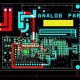# What is LCR Meter & Its Working?

In this tutorial we are going to learn about what is LCR Meter & Its Working?

LCR Meter:

LCR meter is an instrument which measures resistance, inductance as well as capacitance. The main component of LCR meter is the Wheatstone bridge   And RC ratio arm circuits. The component whose value is to be measured is connected in one of the bridge. There are different provisions for the different type of measurement. For example, if the value of resistance is to be measured, then Wheatstone bridge comes into picture while the value of inductance and capacitance can be measured by comparing it with standard capacitor in RC ratio arm circuit. As per above block diagram clearly defines the connection diagram of the LCR meter. In LCR meter for The measurement of DC quantities will be done by exciting the bridge with DC voltage. On the contrary, the AC measurements require excitation of the Wheatstone bridge with AC signal.

Working of LCR Meter

The bridge is adjusted is adjusted in null position in order to balance it completely. Besides, the sensitivity of the meter should also be adjusted along with balancing of the bridge is fed to emitter follower circuit. As per block diagram the output from emitter follower circuit is given as an input to detector amplifier. The significance of detector amplifier can be understood by the fact that if the measuring signal is low in magnitude  ,it will not be able to move the indicator of PMMC meter. Thus, in order to achieve the sustainable indication we need to have a high magnitude measuring signal. But it is often observed that while dealing with the measurement process, the magnitude of then measuring signal falls down due to attenuation factor. The problem to this solution is to utilize an amplifier. The rectifier is used in circuit to convert the Ac signal. When the bridge is provided with AC excitation then at the output end of the bridge with  AC  signal needs transformation into DC signal. The component which is to be measured is placed across the test terminals of LCR meter, after which according to the type of component the measurement is performed Inductor impedance is a combination of resistance and reactance so then where Inductors reactance is 2 times pi times frequency times the inductance an LCR. Frequency times the inductance an LCR meter measures the impedance with an AC Signal to determine reactance by the way when I say ac I small signal ac and when I say ac I mean small signal AC and not mains coming out of the wall when measuring a component you can think of a DMM as making DC measurement and an LCR meter making AC measurements to illustrate that difference let’s look at illustrate that difference look at their measurements on an oscilloscope mode on scope screen we see a saw tooth and  these I know this the capacitance of the measured waveform goes up until microfarad causing the meter to change range so now I’ll make some adjustments